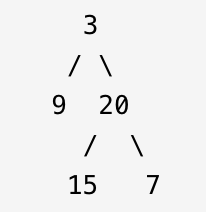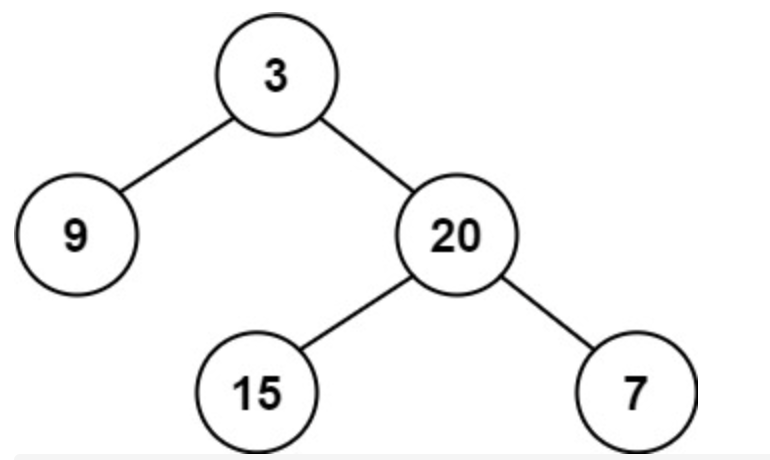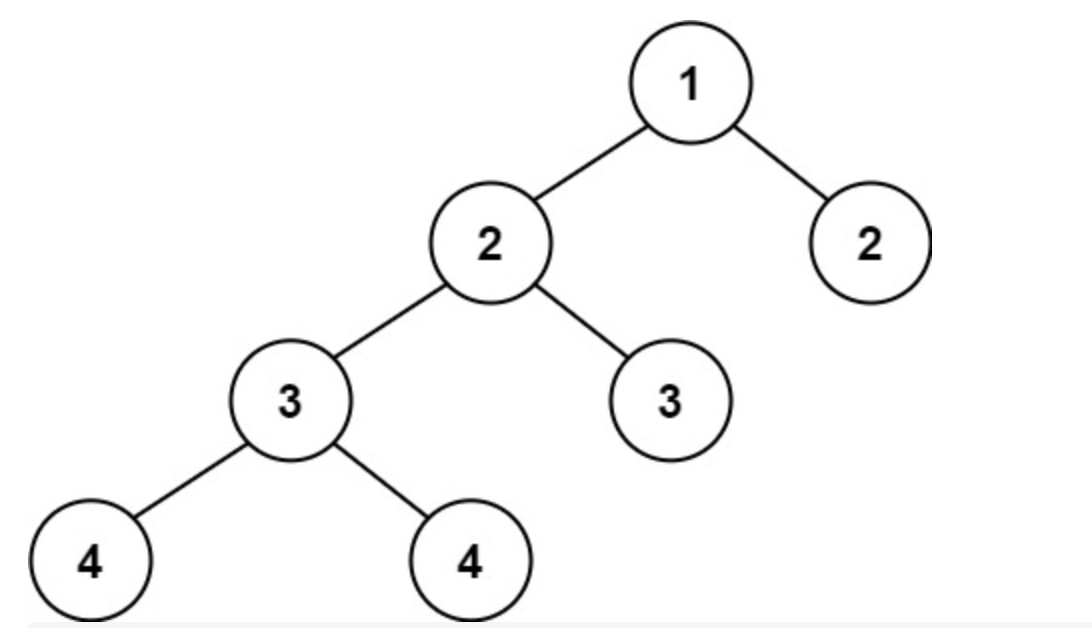• 二叉树的最大深度//平衡二叉树
二叉树的最大深度
int maxDepth(struct TreeNode* root){
//左右子树最大高度 + 根
int left, right;
if (root){
return (left = maxDepth(root->left) + 1) > (right = maxDepth(root->right) + 1) ?
left : right;
}
else{
return 0;
}
}

平衡二叉树
bool _isBalanced(struct TreeNode* root, int* cur){
if (root == NULL){
//当前根节点的高度
*cur = 0;
return true;
}
//下一层能够访问本层的left right
int left = 0, right = 0;
if (_isBalanced(root->left, &left) &&
_isBalanced(root->right, &right) &&
abs(left - right) < 2){
//以当前节点为根的树的最大深度
*cur = left > right ? left + 1 : right + 1;
return true;
}
else{
return false;
}
}
bool isBalanced(struct TreeNode* root){
//根的左右子树, 左右子树的左右子树 是否平衡
int dep = 0;
return _isBalanced(root, &dep);
}

展开全文• 文章目录二叉树最大深度题目描述具体代码1.递归写法2.精简版的递归写法3. 二叉树最大深度 题目描述 输入一棵二叉树的根节点，求该树的深度。从根节点到叶节点依次经过的节点（含根、叶节点）形成树的一条路径，...


文章目录
二叉树的最大深度题目描述具体代码1.递归写法2.精简版的递归写法3.利用层序遍历

平衡二叉树题目描述具体代码

二叉树的最大深度
题目描述
输入一棵二叉树的根节点，求该树的深度。从根节点到叶节点依次经过的节点（含根、叶节点）形成树的一条路径，最长路径的长度为树的深度。
示例 给定二叉树 [3,9,20,null,null,15,7]返回它的最大深度 3 。
具体代码
1.递归写法
代码如下（示例）：
/**
* Definition for a binary tree node.
* struct TreeNode {
*     int val;
*     TreeNode *left;
*     TreeNode *right;
*     TreeNode(int x) : val(x), left(NULL), right(NULL) {}
* };
*/
class Solution {
public:
int getDepth(TreeNode* node){
if (node == NULL) return 0;
int leftdepth = getDepth(node -> left);
int rightdepth = getDepth(node -> right);
int depth = max(leftdepth,rightdepth) + 1;
return depth;
}
int maxDepth(TreeNode* root) {
return getDepth(root);
}
};

2.精简版的递归写法
代码如下（示例）：
class Solution {
public:
int maxDepth(TreeNode* root) {
if (root == NULL) return 0;
return 1 + max(maxDepth(root->left), maxDepth(root->right));
}
};

3.利用层序遍历
代码如下（示例）：
class Solution {
public:
int maxDepth(TreeNode* root) {
queue<TreeNode*> que;
if (root == NULL) return 0;
if (root != NULL) que.push(root);
int level = 0;
while (que.empty() == false){
int size = que.size();
for (int i = 0; i < size; i++){
TreeNode* tem = que.front();
que.pop();
if (tem -> left != NULL) que.push(tem -> left);
if (tem -> right != NULL) que.push(tem -> right);
}
level++;
}
return level;
}
};

平衡二叉树
题目描述
给定一个二叉树，判断它是否是高度平衡的二叉树。 本题中，一棵高度平衡二叉树定义为： 一个二叉树每个节点 的左右两个子树的高度差的绝对值不超过 1 。
示例1输入：root = [3,9,20,null,null,15,7] 输出：true
示例2输入：root = [1,2,2,3,3,null,null,4,4] 输出：false
具体代码
class Solution {
public:
int getheight(TreeNode* node){
if (node == nullptr) return 1;
int leftheight = getheight(node -> left);
int rightheight = getheight(node -> right);
int height = max(leftheight,rightheight) + 1;
return height;
}

bool isBalanced(TreeNode* root) {
if (root == nullptr) return true;
else return abs(getheight(root -> left)-getheight(root -> right)) <= 1 && isBalanced(root -> left) && isBalanced(root -> right);
}
};


展开全文leetcode
• 104. 二叉树最大深度 题目 104. 二叉树最大深度 给定一个二叉树，找出其最大深度二叉树的深度为根节点到最远叶子节点的最长路径上的节点数。 说明: 叶子节点是指没有子节点的节点。 示例： 给定二叉树 [3,9,...
104. 二叉树的最大深度
题目
104. 二叉树的最大深度
给定一个二叉树，找出其最大深度。
二叉树的深度为根节点到最远叶子节点的最长路径上的节点数。
说明: 叶子节点是指没有子节点的节点。
示例： 给定二叉树 [3,9,20,null,null,15,7]， 3 /  9 20 /  15 7 返回它的最大深度 3 。
解法
方法一：深度优先搜索
如果我们知道了左子树和右子树的最大深度 l 和 r，那么该二叉树的最大深度即为 max(l,r)+1
而左子树和右子树的最大深度又可以以同样的方式进行计算。因此我们可以用「深度优先搜索」的方法来计算二叉树的最大深度。具体而言，在计算当前二叉树的最大深度时，可以先递归计算出其左子树和右子树的最大深度，然后在 O(1)时间内计算出当前二叉树的最大深度。递归在访问到空节点时退出。public int maxDepth(TreeNode root) {
if (root == null) {
return 0;
}
return Math.max(maxDepth(root.left), maxDepth(root.right)) + 1;
}

方法二：广度优先搜索
我们也可以用「广度优先搜索」的方法来解决这道题目，但我们需要对其进行一些修改，此时我们广度优先搜索的队列里存放的是「当前层的所有节点」。
每次拓展下一层的时候，不同于广度优先搜索的每次只从队列里拿出一个节点，我们需要将队列里的所有节点都拿出来进行拓展，这样能保证每次拓展完的时候队列里存放的是当前层的所有节点，即我们是一层一层地进行拓展，最后我们用一个变量ans 来维护拓展的次数，该二叉树的最大深度即为ans。
public int maxDepth(TreeNode root) {
if (root == null) {
return 0;
}
int ans = 0;
while (!queue.isEmpty()) {
int size = queue.size();
// 把这一层的都依次取出
while (size-- > 0) {
TreeNode node = queue.remove();
if (node.left != null) {
}
if (node.right != null) {
}
}
ans++;
}
return ans;
}

111. 二叉树的最小深度
题目
111. 二叉树的最小深度
给定一个二叉树，找出其最小深度。
最小深度是从根节点到最近叶子节点的最短路径上的节点数量。
说明：叶子节点是指没有子节点的节点。输入：root = [3,9,20,null,null,15,7] 输出：2 示例 2：
输入：root = [2,null,3,null,4,null,5,null,6] 输出：5
解法
深度优先搜索
叶子节点的定义是左孩子和右孩子都为 null 时叫做叶子节点 当 root 节点左右孩子都为空时，返回 1 当 root 节点左右孩子有一个为空时，返回不为空的孩子节点的深度 当 root 节点左右孩子都不为空时，返回左右孩子较小深度的节点值
public int minDepth(TreeNode root) {
if(root == null) return 0;
//这道题递归条件里分为三种情况
//1.左孩子和有孩子都为空的情况，说明到达了叶子节点，直接返回1即可
if(root.left == null && root.right == null) return 1;
//2.如果左孩子和由孩子其中一个为空，那么需要返回比较大的那个孩子的深度
int m1 = minDepth(root.left);
int m2 = minDepth(root.right);
//这里其中一个节点为空，说明m1和m2有一个必然为0，所以可以返回m1 + m2 + 1;
if(root.left == null || root.right == null) return m1 + m2 + 1;

//3.最后一种情况，也就是左右孩子都不为空，返回最小深度+1即可
return Math.min(m1,m2) + 1;
}

广度优先搜索
同样，我们可以想到使用广度优先搜索的方法，遍历整棵树。
当我们找到一个叶子节点时，直接返回这个叶子节点的深度。广度优先搜索的性质保证了最先搜索到的叶子节点的深度一定最小。
public class Solution {

class QueueNode {
TreeNode node;
int depth;
public QueueNode(TreeNode node, int depth) {
this.node = node;
this.depth = depth;
}
}

public int minDepth(TreeNode root) {
if (root == null) {
return 0;
}
while (!queue.isEmpty()) {
QueueNode nodeDepth = queue.remove();
TreeNode node = nodeDepth.node;
int depth = nodeDepth.depth;
if (node.left == null && node.right == null) {
return depth;
}
if (node.left != null) {
}
if (node.right != null) {
}
}
return 0;
}
}

559. N 叉树的最大深度
题目
559. N 叉树的最大深度
给定一个 N 叉树，找到其最大深度。
最大深度是指从根节点到最远叶子节点的最长路径上的节点总数。
N 叉树输入按层序遍历序列化表示，每组子节点由空值分隔（请参见示例）输入：root = [1,null,3,2,4,null,5,6] 输出：3
解答
深度优先搜索
解决这个问题的最直观方法就是递归。 递归去求每个子节点的最大深度，返回其中最大的子树深度+1 不同的是，二叉树中求的是左子树和右子树的最大值。
public int maxDepth(Node root) {
if (root == null) {
return 0;
}
// 如果没有子树 则为1
if (root.children.isEmpty()) {
return 1;
}
// 递归去求每个子节点的最大深度
List<Integer> heights = new ArrayList<>();
for (Node item : root.children) {
}
// 返回其中最大的深度+1
return Collections.max(heights) + 1;
}

迭代
我们也可以用「广度优先搜索」的方法来解决这道题目，但我们需要对其进行一些修改，此时我们广度优先搜索的队列里存放的是「当前层的所有节点」。
每次拓展下一层的时候，不同于广度优先搜索的每次只从队列里拿出一个节点，我们需要将队列里的所有节点都拿出来进行拓展，这样能保证每次拓展完的时候队列里存放的是当前层的所有节点，即我们是一层一层地进行拓展，最后我们用一个变量ans 来维护拓展的次数，该二叉树的最大深度即为ans。
public int maxDepth(Node root) {
if (root == null) {
return 0;
}
int depth = 0;
while (!queue.isEmpty()) {
int n = queue.size();
depth++;
// 每一层的所有节点都遍历一遍
while (n-- != 0) {
// 所有子节点都入队
for (Node item : queue.remove().children) {
}
}
}
return depth;
}

110. 平衡二叉树
题目
110. 平衡二叉树
给定一个二叉树，判断它是否是高度平衡的二叉树。
本题中，一棵高度平衡二叉树定义为：
一个二叉树每个节点 的左右两个子树的高度差的绝对值不超过 1 。输入：root = [1,2,2,3,3,null,null,4,4] 输出：false
解法
从底至顶（提前阻断）
此方法为本题的最优解法，但“从底至顶”的思路不易第一时间想到。
思路是对二叉树做先序遍历，从底至顶返回子树最大高度，若判定某子树不是平衡树则 “剪枝” ，直接向上返回。
算法流程：
recur(root):
递归返回值：
当节点root 左 / 右子树的高度差 < 2：则返回以节点root为根节点的子树的最大高度，即节点 root 的左右子树中最大高度加 1（ max(left, right) + 1 ）；
当节点root 左 / 右子树的高度差 ≥2 ：则返回 −1 ，代表 此子树不是平衡树 。  递归终止条件：
当越过叶子节点时，返回高度 0 ；
当左（右）子树高度 left== -1 时，代表此子树的 左（右）子树 不是平衡树，因此直接返回 -1 ；
	public boolean isBalanced(TreeNode root) {
return recur(root) != -1;
}

public int recur(TreeNode root) {
// 叶子节点高度为0
if (root == null) {
return 0;
}
// 如果不平衡则直接返回
int leftHeight = recur(root.left);
if (leftHeight == -1) {
return -1;
}
int rightHeight = recur(root.right);
if (rightHeight == -1) {
return -1;
}
// 判断是否高度相差在2以内
return Math.abs(leftHeight - rightHeight) < 2 ? Math.max(leftHeight, rightHeight) + 1 : -1;
}

从顶至底（暴力法）
此方法容易想到，但会产生大量重复计算，时间复杂度较高。
思路是构造一个获取当前节点最大深度的方法 depth(root) ，通过比较此子树的左右子树的最大高度差abs(depth(root.left) - depth(root.right))，来判断此子树是否是二叉平衡树。若树的所有子树都平衡时，此树才平衡。
class Solution {
public boolean isBalanced(TreeNode root) {
if (root == null) return true;
return Math.abs(depth(root.left) - depth(root.right)) <= 1 && isBalanced(root.left) && isBalanced(root.right);
}

private int depth(TreeNode root) {
if (root == null) return 0;
return Math.max(depth(root.left), depth(root.right)) + 1;
}
}

展开全文• 104. 二叉树最大深度 给定一个二叉树，找出其最大深度二叉树的深度为根节点到最远叶子节点的最长路径上的节点数。 说明: 叶子节点是指没有子节点的节点。 示例： 给定二叉树 [3,9,20,null,null,15,7]， 3 / \...
前序遍历和后序遍历的代码区别
前序的递归函数的 返回值为空；参数部分含有结果值；return；后续的递归函数的 返回值为求值的类型； 参数部分不含有结构值； return 类型+1；
104. 二叉树的最大深度
给定一个二叉树，找出其最大深度。
二叉树的深度为根节点到最远叶子节点的最长路径上的节点数。
说明: 叶子节点是指没有子节点的节点。
示例：
给定二叉树 [3,9,20,null,null,15,7]，
3
/ \
9  20
/  \
15   7
返回它的最大深度 3 。

递归法思路：求最大深度，应该是前序遍历，但是最大深度也可以是高度，因此用后序遍历也可以
int maxDepth(TreeNode* root){
if(root==NULL) return 0;
return getDepth(root);
}
int getDepth(TreeNode* cur){
if(cur==NULL) return 0;
int leftdepth=getDepth(cur->left);
int rightdepth=getDepth(cur->right);
return max(leftdepth,rightdepth)+1;
}

迭代法思路：层序遍历最适合，因为遍历的次数就是层数就是最大深度
  int maxDepth(TreeNode* root) {//层序遍历
queue<TreeNode*> que;
int depth=0;
if(root!=NULL) que.push(root);
while(!que.empty()){
int size=que.size();
depth++;
for(int i=0;i<size;i++){
TreeNode* cur=que.front();
que.pop();
if(cur->left) que.push(cur->left);
if(cur->right) que.push(cur->right);
}
}
return depth;
}

559. N叉树的最大深度
给定一个 N 叉树，找到其最大深度。

最大深度是指从根节点到最远叶子节点的最长路径上的节点总数。

例如，给定一个 3叉树 :

我们应返回其最大深度，3。

 int maxDepth(Node* root) {//层序遍历
queue<Node*> que;
int depth=0;
if(root!=NULL) que.push(root);
while(!que.empty()){
int size=que.size();
depth++;
for(int i=0;i<size;i++){
Node* cur=que.front();
que.pop();
for(int j=0;j<cur->children.size();j++){
if(cur->children[j]) que.push(cur->children[j]);
}
}
}
return depth;
}

 int maxDepth(Node* root) {//递归
return getmax(root);
}
int getmax(Node* node){
if(node==NULL) return 0;
int depth=0;
for(int i=0;i<node->children.size();i++){
depth=max(depth,getmax(node->children[i]));
}
return depth+1;
}

111. 二叉树的最小深度
给定一个二叉树，找出其最小深度。
最小深度是从根节点到最近叶子节点的最短路径上的节点数量。
说明: 叶子节点是指没有子节点的节点。
示例:
给定二叉树 [3,9,20,null,null,15,7],
3
/ \
9  20
/  \
15   7

迭代思路：比较好想的是用层序遍历来遍历结点，判断每一个结点是不是叶结点，如果是叶结点，那么就结束遍历，当前层数就是最小深度
 int minDepth(TreeNode* root) {
int depth=0;
queue<TreeNode*> que;
if(root!=NULL) que.push(root);
while(!que.empty()){
int size=que.size();
depth++;
for(int i=0;i<size;i++){
TreeNode* cur=que.front();
que.pop();
if(cur->left) que.push(cur->left);
if(cur->right) que.push(cur->right);
if(cur->left==NULL&&cur->right==NULL) return depth;
}
}
return depth;
}

递归思路：和求最大深度的思路相似，但是需要注意的地方在于，当根结点的左结点或者右结点为空的时候，min()为0，但其实最小深度不为0，而是另外一个子树的最小深度。 错误：
int minDepth(TreeNode* root) {

}
int getDepth(TreeNode* cur){
if(cur==NULL) return 0;
int left=getDepth(cur->left);
int right=grtDepth(cur->right);
return min(left,right)+1;
}

正确：
int minDepth(TreeNode* root) {
return getDepth(root);
}
int getDepth(TreeNode* cur){
if(cur==NULL) return 0;
if(cur->left==NULL&&cur->right!=NULL) return 1+getDepth(cut->right);
if(cur->right==NULL&&cur->left!=NULL) return 1+getDepth(cut->left);

int left=getDepth(cur->left);
int right=grtDepth(cur->right);
return min(left,right)+1;
}

222. 完全二叉树的节点个数
给出一个完全二叉树，求出该树的节点个数。

说明：

完全二叉树的定义如下：在完全二叉树中，除了最底层节点可能没填满外，其余每层节点数都达到最大值，并且最下面一层的节点都集中在该层最左边的若干位置。若最底层为第 h 层，则该层包含 1~ 2h 个节点。

示例:

输入:
1
/ \
2   3
/ \  /
4  5 6

输出: 6

递归思路：左边结点个数加上右边结点个数
class Solution1 {//递归
public:
int countNodes(TreeNode* root) {
return getNodeNum(root);
}
int getNodeNum(TreeNode* root){
if(root==NULL) return 0;
int leftNum=getNodeNum(root->left);
int rightNum=getNodeNum(root->right);
return leftNum+rightNum+1;
}
};


迭代思路；层序遍历统计每层个数
class Solution {//迭代
public:
int countNodes(TreeNode* root) {
int num=0;
queue<TreeNode*> que;
if(root!=NULL) que.push(root);
while(!que.empty()){
int size=que.size();
num+=size;
for(int i=0;i<size;i++){
TreeNode* cur=que.front();
que.pop();
if(cur->left) que.push(cur->left);
if(cur->right) que.push(cur->right);
}
}
return num;
}
};

110. 平衡二叉树
给定一个二叉树，判断它是否是高度平衡的二叉树。
本题中，一棵高度平衡二叉树定义为：
一个二叉树每个节点 的左右两个子树的高度差的绝对值不超过1。
示例 1:
给定二叉树 [3,9,20,null,null,15,7]
3
/ \
9  20
/  \
15   7
返回 true 。

示例 2:
给定二叉树 [1,2,2,3,3,null,null,4,4]

1
/ \
2   2
/ \
3   3
/ \
4   4
返回 false 。

递归思路：比较每一个结点的高度，显然用后序遍历，比较当前结点的左右子树的最大高度，大于1则不平衡 不容易想到的一点是，如果差大于1就不应返回高度，而是返回一个不可能的值，主函数判断是不是这个值，如果是则就返回false；
 bool isBalanced(TreeNode* root) {
if(root==NULL) return true;
return getdiff(root)==-1?false:true;
}
int getdiff(TreeNode* cur){
if(cur==NULL) return 0;
int left=getdiff(cur->left);
if(left==-1){return -1;}
int right=getdiff(cur->rigth);
if(right==-1){return -1;}
//当结点的差不为1时，就不用返回高度了；
return abs(left-right)>1?-1:max(left,right)+1;
}

展开全文• 本题中，一棵高度平衡二叉树定义为： 一个二叉树每个节点的左右两个子树的高度差的绝对值不超过1。 给定二叉树[3,9,20,null,null,15,7] 3 / \ 9 20 / \ 15 7 返回true。示例 2: 给定二叉树[1,2,2,3,3,n...
• 二叉树深度：根节点到当前节点的节点数 故求高度可用后序遍历，而深度可用前序遍历 class Solution { public: // 返回以该节点为根节点的二叉树的高度，如果不是二叉搜索树了则返回-1 int getDepth(TreeNode* ...
• 一棵高度平衡二叉树定义为：一个二叉树每个节点 的左右两个子树的高度差的绝对值不超过 1 。 示例1： 输入：root = [3,9,20,null,null,15,7] 输出：true 示例2： 输入：root = [1,2,2,3,3,null,null,4,4] 输出：...leetcode 算法
• 104. 二叉树的最大深度 110. 平衡二叉树 104. 二叉树的最大深度 【题目】： 【代码】： 效果： 110. 平衡二叉树 【题目】： 给定一个二叉树，判断它是否是高度平衡的二叉树。 本题中，一棵高度平衡...
• 平衡二叉树 题解 二叉树的深度（后序遍历、层序遍历，清晰图解） 平衡二叉树（从底至顶、从顶至底，清晰图解） 思路 二叉树的深度 平衡二叉树 代码 二叉树的深度 # Definition for a binary tree ...
• 文章目录一、 二叉树结构体一、求最大深度 一、 二叉树结构体 /*** * 二叉树基本的方法 */ public class TreeNode { private String val; private TreeNode left; private TreeNode right; TreeNode...
• 104. 二叉树最大深度 给定一个二叉树，找出其最大深度二叉树的深度为根节点到最远叶子节点的最长路径上的节点数。 说明: 叶子节点是指没有子节点的节点。 示例： 给定二叉树 [3,9,20,null,null,15,7] 3 ...
• 输入一棵二叉树，求该树的深度。从根结点到叶结点依次经过的结点（含根、叶结点）形成树的一条路径，最长路径的长度为树的深度--一个根节点的左右有几个子节点，而该树的深度就是求左右子节点的最大一个+1public int...二叉树
• 平衡二叉树的定义：要求每个结点的左右差为0或者-1或者1； 方法：嵌套法 树空时，n0=0; 第一层n1=1; 公式：N_h=N_h-1+N_h-2+1; 第二层n2=1+0+1=2; 第三层n3=2+1+1=4; 第四层n4=4+2+1=7; 第五层n5=7+4+1=12; 因此，...数据结构
• 104. 二叉树最大深度 给定一个二叉树，找出其最大深度二叉树的深度为根节点到最远叶子节点的最长路径上的节点数。 说明: 叶子节点是指没有子节点的节点。 思路 直接DFS，返回左右子树深度大的即可。 代码 /** ...
• 二叉树最大深度 给定一个二叉树，找出其最大深度二叉树的深度为根节点到最远叶子节点的最长路径上的节点数。 说明: 叶子节点是指没有子节点的节点。 示例： 给定二叉树 [3,9,20,null,null,15,7] 3 / \ 9 ...算法 数据结构
• 如果某二叉树中任意结点的左右子树的深度相差不超过1，那么它就是一棵平衡二叉树。 输入：二叉树[5,7,11,null,null,12,9,null,null,null,null]如下所示， 5 / \ 7 11 / \ 12 9 输出：true    思路：二叉树...数据结构...